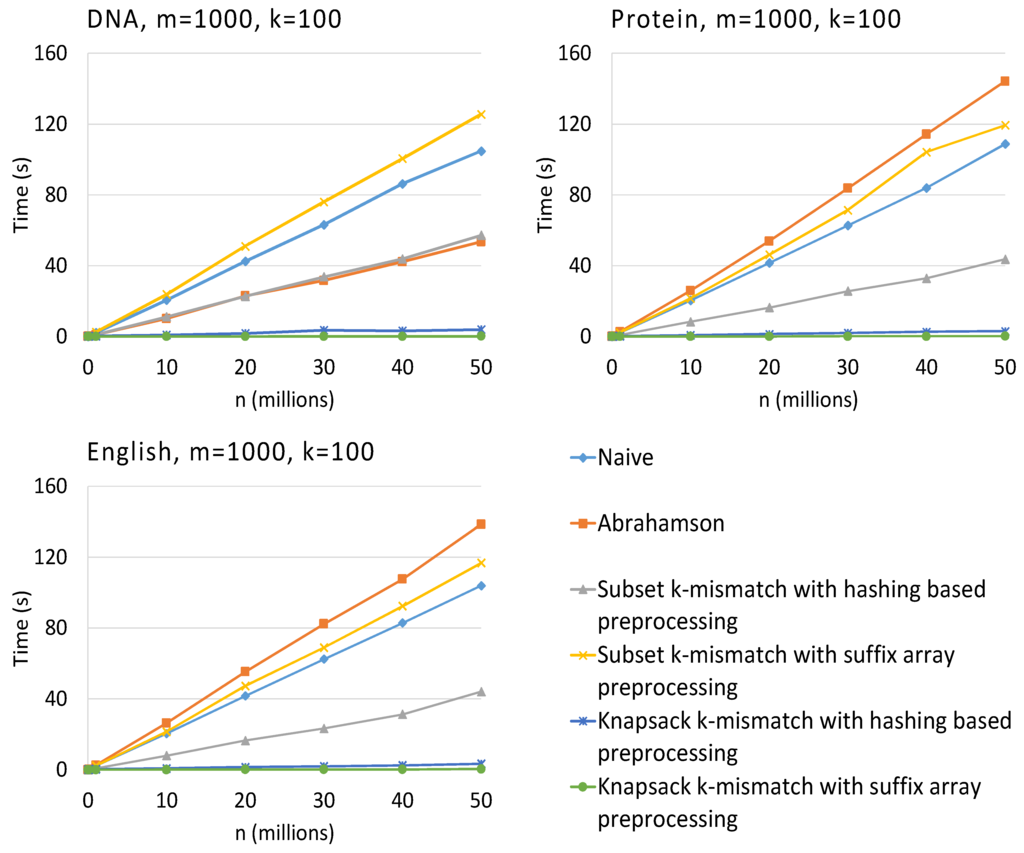Next Article in Journal
Dynamics and Fractal Dimension of Steffensen-Type Methods
Next Article in Special Issue
Modified Classical Graph Algorithms for the DNA Fragment Assembly Problem
Previous Article in Journal
An Optimization Clustering Algorithm Based on Texture Feature Fusion for Color Image Segmentation
Open AccessArticle

# On String Matching with Mismatches

Department of Computer Science and Engineering, University of Connecticut, 371 Fairfield Way Unit 4155, Storrs, CT 06269, USA
*
Author to whom correspondence should be addressed.
Academic Editor: Giuseppe Lancia
Algorithms 2015, 8(2), 248-270; https://doi.org/10.3390/a8020248
Received: 3 April 2015 / Accepted: 19 May 2015 / Published: 26 May 2015
(This article belongs to the Special Issue Algorithmic Themes in Bioinformatics)
In this paper, we consider several variants of the pattern matching with mismatches problem. In particular, given a text $$T=t_1 t_2\cdots t_n$$ and a pattern $$P=p_1p_2\cdots p_m$$, we investigate the following problems: (1) pattern matching with mismatches: for every $$i, 1\leq i \leq n-m+1$$ output, the distance between $$P$$ and $$t_i t_{i+1}\cdots t_{i+m-1}$$; and (2) pattern matching with $$k$$ mismatches: output those positions $$i$$ where the distance between $$P$$ and $$t_i t_{i+1}\cdots t_{i+m-1}$$ is less than a given threshold $$k$$. The distance metric used is the Hamming distance. We present some novel algorithms and techniques for solving these problems. We offer deterministic, randomized and approximation algorithms. We consider variants of these problems where there could be wild cards in either the text or the pattern or both. We also present an experimental evaluation of these algorithms. The source code is available at http://www.engr.uconn.edu/$$\sim$$man09004/kmis.zip. View Full-Text
Show FiguresFigure 1

MDPI and ACS Style

Nicolae, M.; Rajasekaran, S. On String Matching with Mismatches. Algorithms 2015, 8, 248-270.

Show more citation formats Show less citations formats

### Article Access Map

1
Only visits after 24 November 2015 are recorded.